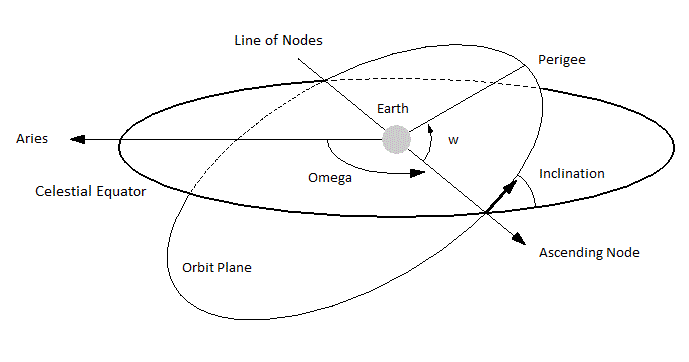# What is Satellite

Satellite – A satellite is a celestial object which revolves around a planet in circular path or elliptical path.There are two types of satellite: – natural satellites and man-made satellites (or artificial satellites). An example of a natural satellite is a moon which revolves around the earth. Now days, the term satellite is used for man-made or artificial satellite which keep on revolving around the earth. An example of man-made or artificial satellite is INSAT-1B. The satellites revolve around the earth which is in a definite path. The closed path of a satellite around the earth is called its orbit of a satellite. The orbit of a satellite can be circular or elliptical in shape.

The various characteristics which define the orbit of a satellite around the earth are1. Apogee – It is the farthest point from the earth on the orbit of a satellite.
2. Perigee – It is the nearest point from the earth on the orbit of a satellite.
3. Inclination – The inclination of the orbit of a satellite is the angle made by the orbit of the satellite with the equator of the earth